Question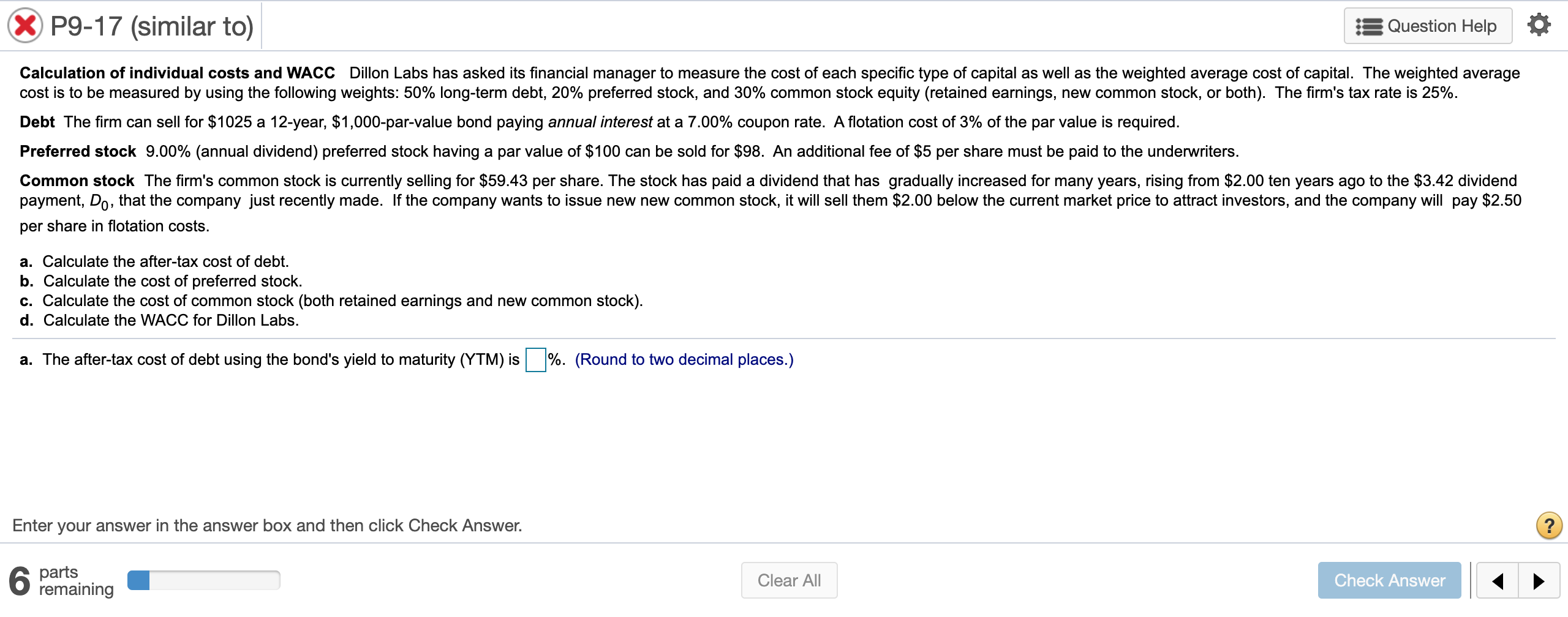a.  The​ after-tax cost of debt using the​ bond's yield to maturity​ (YTM) is

The​ after-tax cost of debt using the approximation formula is

b.  The cost of preferred stock is

c.  The cost of retained earnings is

The cost of new common stock is

d.  Using the cost of retained​ earnings, the​ firm's WACC is

Using the cost of new common​ stock, the​ firm's WACC is

The​ after-tax cost of debt using the approximation formula is:-

=(70+((1000-994.25)/12))/((1000+994.25)/2)*(1-25%) =5.30%

Cost of retained earnings approach:-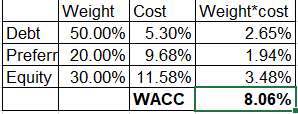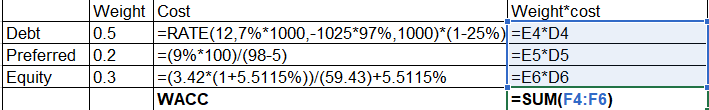Cost of equity approach:-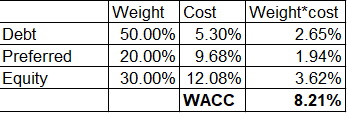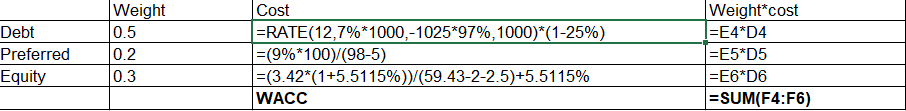The growth rate of dividend is calculated using the RATE function as follows:-

=RATE(nper,pmt,pv,fv)

=RATE(10,,-2,3.42)

=5.115%

#### Earn Coins

Coins can be redeemed for fabulous gifts.

Similar Homework Help Questions
• ### A. Calculate the after-tax cost of debt. b. Calculate the cost of preferred stock. c. Calculate t...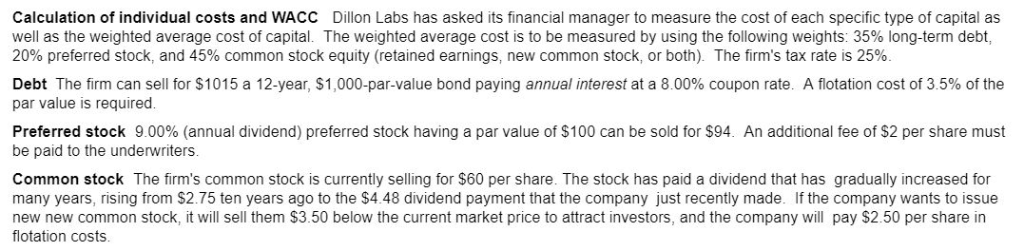a. Calculate the after-tax cost of debt. b. Calculate the cost of preferred stock. c. Calculate the cost of common stock (both retained earnings and new common stock). d. Calculate the WACC for Dillon Labs. Calculation of individual costs and WACC Dillon Labs has asked its financial manager to measure the cost of each specific type of capital as well as the weighted average cost of capital. The weighted average cost is to be measured by using the following weights:...

• ### Need assistance with A, B, C, D Calculation of individual costs and WACC Dillon Labs has...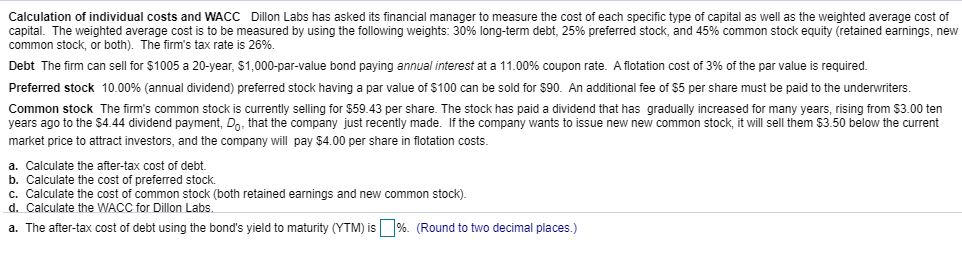Need assistance with A, B, C, D Calculation of individual costs and WACC Dillon Labs has asked its financial manager to measure the cost of each specific type of capital as well as the weighted average cost of capital. The weighted average cost is to be measured by using the following weights: 30% long-term debt, 25% preferred stock, and 45% common stock equity (retained earnings, new common stock, or both). The firm's tax rate is 26%. Debt The firm can...

• ### Please answer all parts of the question, thank you to anyone who helps me out! P9-17...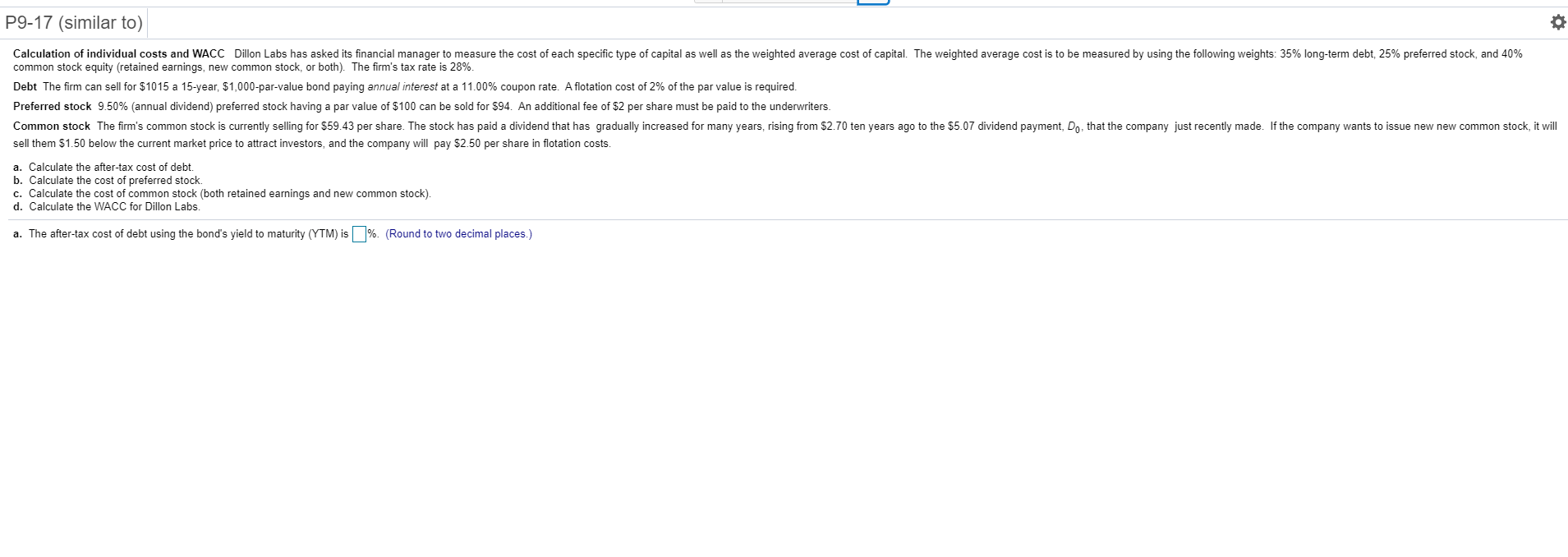Please answer all parts of the question, thank you to anyone who helps me out! P9-17 (similar to) 0 Calculation of individual costs and WACC Dillon Labs has asked its financial manager to measure the cost of each specific type of capital as well as the weighted average cost of capital. The weighted average cost is to be measured by using the following weights: 35% long-term debt, 25% preferred stock, and 40% common stock equity (retained earnings, new common stock,...

• ### Calculation of individual costs and WACC Dillon Labs has asked its financial manager to measure the...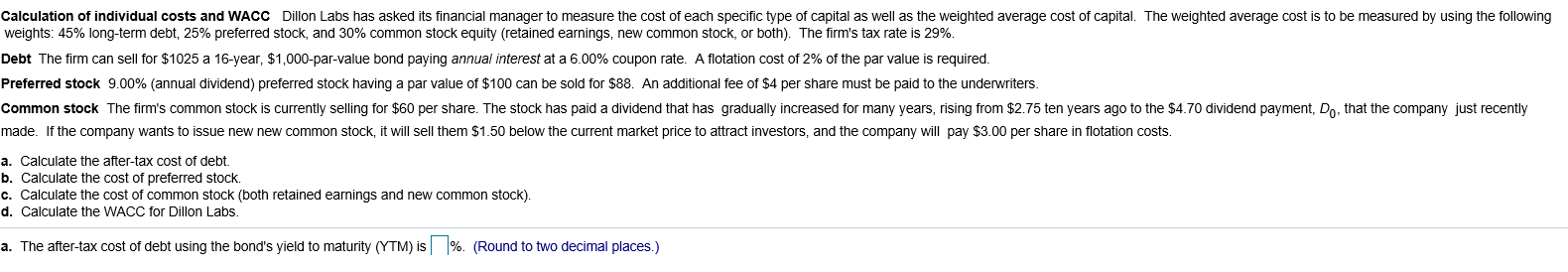Calculation of individual costs and WACC Dillon Labs has asked its financial manager to measure the cost of each specific type of capital as well as the weighted average cost of capital. The weighted average cost is to be measured by using the following weights: 45% long-term debt, 25% preferred stock, and 30% common stock equity (retained earnings, new common stock, or both). The firm's tax rate is 29%. Debt The firm can sell for \$1025 a 16-year, \$1,000-par-value bond...

• ### P9-17 (book/static) s Question Help Calculation of individual costs and WACC Dillon Labs has asked its...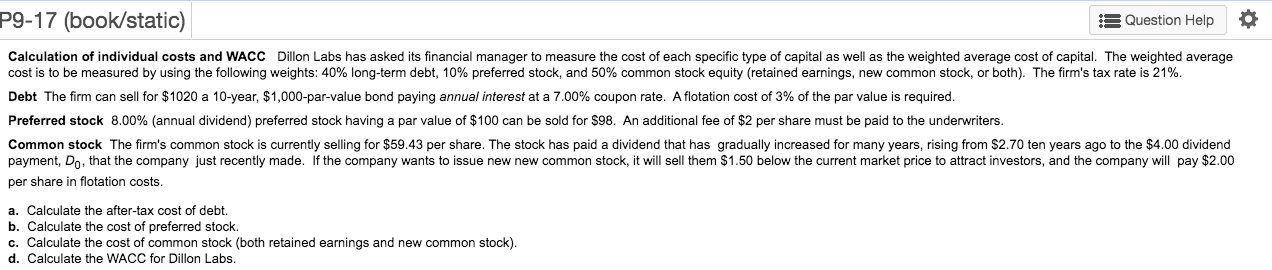P9-17 (book/static) s Question Help Calculation of individual costs and WACC Dillon Labs has asked its financial manager to measure the cost of each specific type of capital as well as the weighted average cost of capital. The weighted average cost is to be measured by using the following weights: 40% long-term debt, 10% preferred stock, and 50% common stock equity (retained earnings, new common stock, or both). The firm's tax rate is 21%. Debt The firm can sell for...

• ### P9-17 (similar to) Question Help Calculation of individual costs and WACC Dillon Labs has asked its...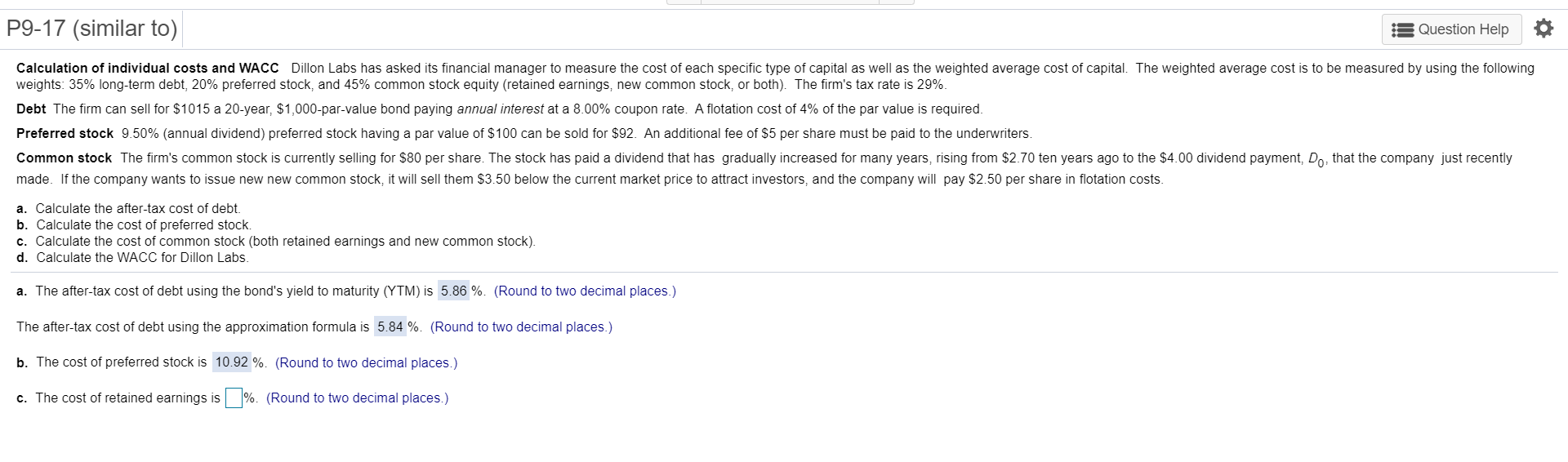P9-17 (similar to) Question Help Calculation of individual costs and WACC Dillon Labs has asked its financial manager to measure the cost of each specific type of capital as well as the weighted average cost of capital. The weighted average cost is to be measured by using the following weights: 35% long-term debt, 20% preferred stock, and 45% common stock equity (retained earnings, new common stock, or both). The firm's tax rate is 29%. Debt The firm can sell for...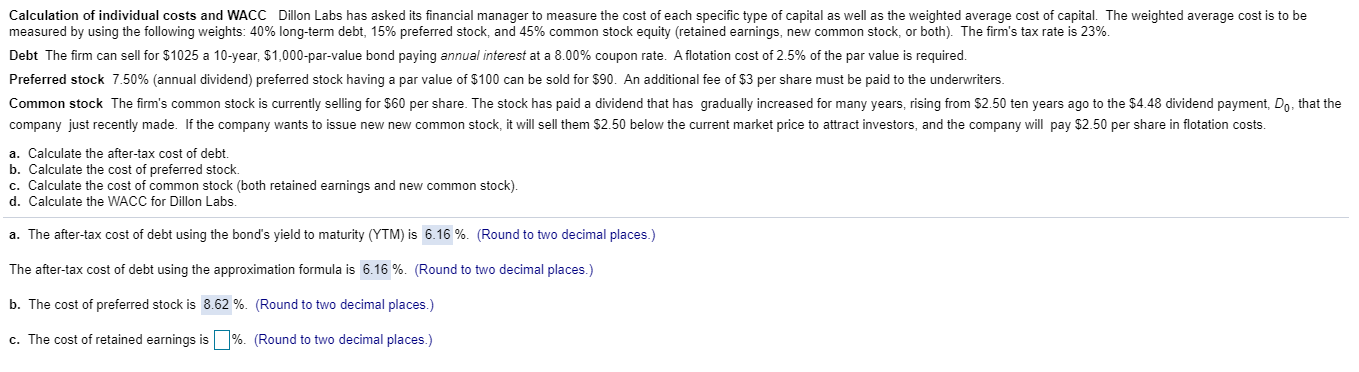Please help with part c & d! Show your work ! Thanks Calculation of individual costs and WACC Dillon Labs has asked its financial manager to measure the cost of each specific type of capital as well as the weighted average cost of capital. The weighted average cost is to be measured by using the following weights: 40% long-term debt, 15% preferred stock, and 45% common stock equity (retained earnings, new common stock, or both). The firm's tax rate is...

• ### Dillon Labs has asked its financial manager to measure the cost of each specific type of...

Dillon Labs has asked its financial manager to measure the cost of each specific type of capital as well as the weighted average cost of capital. The weighted average cost is to be measured by using the following​ weights: 45​% ​long-term debt, 25​% preferred​ stock, and 30​% common stock equity​ (retained earnings, new common​ stock, or​ both). The​ firm's tax rate is 28​%. Debt The firm can sell for ​\$1030 a 16​-year, \$1,000​-par-value bond paying annual interest at a 11.00​%...

• ### P9-17 (similar to) Calculation of individual costs and WACC Dillon Labs has asked its financial manager...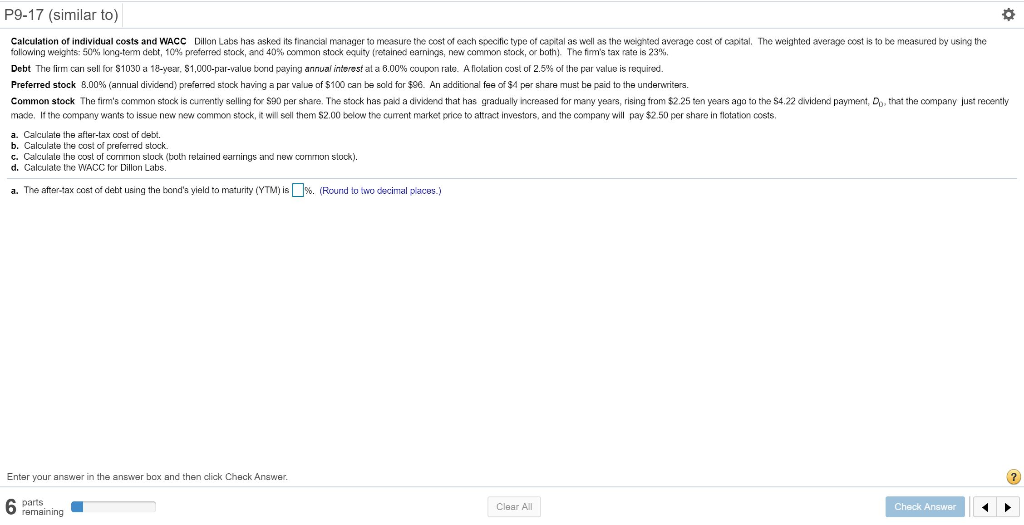P9-17 (similar to) Calculation of individual costs and WACC Dillon Labs has asked its financial manager to measure the cost of each specific type of capital as well as the weighted average cost of capital. The weighted average cost is to be measured by using the following weights: 50% long-term debt, 10% preferred stock, and 40%, common stock equity (retained earnings, new common stock, or both). The firm's tax rate is 23%. Debt The firm can sell for \$1030 a...

• ### Dillon Labs has asked its financial manager to measure the cost of each specific type of capital as well as the weighted...

Dillon Labs has asked its financial manager to measure the cost of each specific type of capital as well as the weighted average cost of capital. The weighted average cost is to be measured by using the following​ weights: 40​% ​long-term debt, 25​% preferred​ stock, and 35​% common stock equity​ (retained earnings, new common​ stock, or​ both). The​ firm's tax rate is 22​%. Debt The firm can sell for ​\$1020 a 20​-year, ​\$1,000​-par-value bond paying annual interest at a 8.00​%...